﻿ DPSFHelper.RandomVectorBetweenTwoVectors Method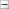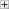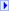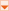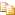Collapse AllExpand AllCode: All Code: Multiple Code: C# Code: Visual Basic Code: Visual C++

[TCheckBoxFormControlObj]C#

[TCheckBoxFormControlObj]Visual Basic

[TCheckBoxFormControlObj]
Visual C++
 DPSF API Documentation DPSFHelper . . :: ..RandomVectorBetweenTwoVectors MethodReturns a Vector whose individual XYZ components are each randomly chosen to be somewhere between the two given Vectors' individual XYZ components. Unlike choosing a random Lerp value between two vectors, which would give a point somewhere on the LINE between the two points, this chooses a random Lerp value between each of the two vectors individual xyz components, returning a point somewhere in the cube-shaped AREA (i.e. Volume) between the two points.

Namespace:

Assembly: DPSF (in DPSF.dll) Version: 2.5.0.0Syntax

 C# public static Vector3 RandomVectorBetweenTwoVectors(  Vector3 sVector1,  Vector3 sVector2 )
 Visual Basic Public Shared Function RandomVectorBetweenTwoVectors (  sVector1 As Vector3,  sVector2 As Vector3 ) As Vector3
 Visual C++ public: static Vector3 RandomVectorBetweenTwoVectors(  Vector3 sVector1,  Vector3 sVector2 )

Parameters

sVector1

Type: Vector3

The first Vector

sVector2

Type: Vector3

The second Vector

Return Value

Type: Vector3

Returns a Vector whose individual XYZ components are each randomly chosen to be somewhere between the two given Vectors' individual XYZ components.See Also

DPSFHelper Class

DPSFHelper Members

DPSF NamespaceSend comments on this topic to